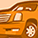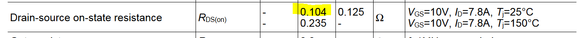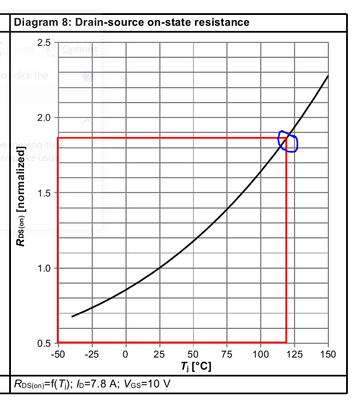Tip / Sign in to post questions, reply, level up, and achieve exciting badges. Know more

# MOSFET (Si/SiC) Forum DiscussionsLevel 1Level 1

# Normalized RDS(on) graph

HI

I have referred to the datasheet of MOSFET IPAN60R125PFD7S.

My question is regarding calculating RDS(on) value at temperature of 120C.

When I refer the graph RSD(on) is mentioned as Normalized.

My questions are :

1) What is the meaning and use of this Normalized graph .

2) How I can calculate RDS(on) value at different temperature (ta = 120)

1 SolutionModeratorModerator

# Re: Normalized RDS(on) graph

hello @SSJP

Thanks for Posting your question in Infineon community.

Q1. What is the meaning and use of this Normalized graph .

Ans. MOSFET RDSon temperature coefficient is positive. That means RDSon will increase with increase in  temperature.

Generally, in datasheet the values of  RDSon will be given at junction temperature of 25degC &  125degC (some times at 150degC).

but in design requirement we may not operate the device junction temperature at either  25degC &  125degC (some times at 150degC).

in this case we need to know the RDSon value at our operating junction temperature. so for that purpose we can use this normalized RDSon with temperature graph to get the temperature coefficient.

Q2. How I can calculate RDS(on) value at different temperature (tj = 120)

Ans. For "MOSFET IPAN60R125PFD7S"  the typical RDSon  at 25degC junction temperature is 0.104 ohms with datasheet conditions as shown in below picture.the corresponding RDSon of the MOSFET at 120degC junction temperature is 0.104 ohm X 1.85 = 0.1924 ohm.so due to increase in junction temperature (from 25degC to 120degC) MOSFET RDSon (positive temperature coefficient) the value of RDSon is also increased from 0.104ohms to 0.1924ohms.

Thanks,

Malleswararao

2 RepliesModeratorModerator

# Re: Normalized RDS(on) graph

hello @SSJP

Thanks for Posting your question in Infineon community.

Q1. What is the meaning and use of this Normalized graph .

Ans. MOSFET RDSon temperature coefficient is positive. That means RDSon will increase with increase in  temperature.

Generally, in datasheet the values of  RDSon will be given at junction temperature of 25degC &  125degC (some times at 150degC).

but in design requirement we may not operate the device junction temperature at either  25degC &  125degC (some times at 150degC).

in this case we need to know the RDSon value at our operating junction temperature. so for that purpose we can use this normalized RDSon with temperature graph to get the temperature coefficient.

Q2. How I can calculate RDS(on) value at different temperature (tj = 120)

Ans. For "MOSFET IPAN60R125PFD7S"  the typical RDSon  at 25degC junction temperature is 0.104 ohms with datasheet conditions as shown in below picture.the corresponding RDSon of the MOSFET at 120degC junction temperature is 0.104 ohm X 1.85 = 0.1924 ohm.so due to increase in junction temperature (from 25degC to 120degC) MOSFET RDSon (positive temperature coefficient) the value of RDSon is also increased from 0.104ohms to 0.1924ohms.

Thanks,

MalleswararaoLevel 1Level 1

# Re: Normalized RDS(on) graph